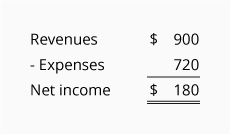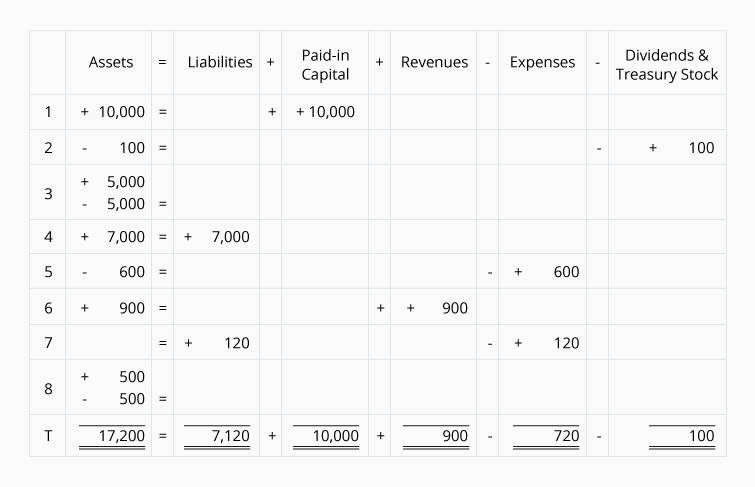# Expanded Accounting Equation for a Sole Proprietorship

The owner's equity in the basic accounting equation is sometimes expanded to show the accounts that make up owner's equity: Owner's Capital, Revenues, Expenses, and Owner's Draws.

Instead of the accounting equation, Assets = Liabilities + Owner's Equity, the expanded accounting equation is:The eight transactions that we had listed under the basic accounting equation Transaction 8, are shown in the following expanded accounting equation:With the expanded accounting equation, you can easily see the company's net income:## Expanded Accounting Equation for a Corporation

The stockholders' equity part of the basic accounting equation can also be expanded to show the accounts that make up stockholders' equity: Paid-in Capital, Revenues, Expenses, Dividends, and Treasury Stock.

Instead of the accounting equation, Assets = Liabilities + Stockholders' Equity, the expanded accounting equation is:The eight transactions that we had listed under the basic accounting equation Transaction C8 are shown in the following expanded accounting equation:With the expanded accounting equation, you can easily see the corporation's net income:#### Take Our Practice Quiz

We recommend that you now take our free Practice Quiz for this topic so that you can...

• See what you know
• See what you don't know